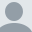# Ask a Jedi: Formatting large numbers

This post is more than 2 years old.

This wasn't technically a 'Ask a Jedi' question but was posted to my forums. The question was - how can we convert numbers into smaller versions? The examples given by the user were:

123,000 to 123K
123,000,000 to 123M
123,000,000,000 to 123B

I checked CFlib of course, and the closest thing I found was ByteConvert. I played around a bit and came up with the following UDF:

`<cfscript> function formatKMB(x) { if(x < 1000) return x; if(x >= 1000 && x < 1000000) { x = x/1000; x = round(x); return x & "K"; } if(x >= 1000000 && x < 1000000000) { x = x/1000000; x = round(x); return x & "M"; } if(x >= 1000000000 && x < 1000000000000) { x = x/1000000000; x = round(x); return x & "B"; } return x; } </cfscript>`

It's probably a bit more verbose then it needs to be but it handles numbers in the thousands (Ks), millions (Ms), and billions (Bs). I wrote up a quick test to see if it worked:

`<cfset tests = "900,1002,1932,123000,432000,1000000,92000000,102000000000,2321903211"> <cfloop index="t" list="#tests#"> <cfoutput> #t#=#formatKMB(t)#<br /> </cfoutput> </cfloop>`

I'll post this to CFLib a bit later. First I'm going to remove the CF8 stuff (<, >) so it will work CF5 and higher.

Raymond Camden's Picture

## About Raymond Camden

Raymond is a senior developer evangelist for Adobe. He focuses on document services, JavaScript, and enterprise cat demos. If you like this article, please consider visiting my Amazon Wishlist or donating via PayPal to show your support. You can even buy me a coffee!

Lafayette, LA

### Archived CommentsComment 1 by Rick O posted on 10/17/2008 at 6:12 AM

Cheat and fall back on your high school math classes:

function byteFormat(b) {
var sign = "";
var suffix = "B";
var groups = 0;
if(b lt 0) { sign = "-"; b = -b; }
if(b neq 0) groups = int(int(log10(b)) / 3);
b = round(b / (1000 ^ groups));
if(groups gt 0) suffix = mid("KMGTEP",groups,1) & suffix;
return sign & b & suffix;
}

For extra credit, make a base-1024 version.Comment 2 by Rick O posted on 10/17/2008 at 6:14 AM

Hmm. I misread your original post and though you were going for byte formatting. Still, the change to your system is pretty straightforward. (Suffix starts empty, then the list of suffixes is "KMB", etc.)Comment 3 by Rick O posted on 10/17/2008 at 6:37 AM

Okay, last post. Really. Cross my heart. How about a version that lets you specify not only the base (such as 1000 or 1024) but also the possible suffixes?

function byteFormat() {
var b = arguments;
var base = 1000;
var sign = "";
var suffix = "B";
var groups = 0;
var suffixes = listToArray("B,KB,MB,GB,TB,PB,EB,ZB,YB");
if(not isNumeric(b)) b = 0;
if((arrayLen(arguments) gte 2) and isNumeric(arguments)) base = int(arguments);
if((arrayLen(arguments) gte 3) and isArray(arguments)) suffixes = arguments;
if(b lt 0) { sign = "-"; b = -b; }
if(b neq 0) groups = min(int(log(b) / log(base)),arrayLen(suffixes)-1);
b = round(b / (base ^ groups));
return sign & numberFormat(b) & suffixes[groups+1];
return groups;
}

Tests:

// negatives work
byteFormat(-12345): -12KB

// zero works
byteFormat(0): 0B

// base-1000 vs base-1024
byteFormat(1000): 1KB
byteFormat(1000,1024): 1,000B
byteFormat(1024,1024): 1KB

// custom suffixes
byteFormat(1024,1024,listToArray("B,KiB")): 1KiB

// numbers that exceed the list of custom suffixes
byteFormat(1234567,1024,listToArray("B,KiB")): 1,206KiBComment 4 by Steve Withington posted on 10/17/2008 at 7:55 AM

Wouldn't something like this be just as easy:

<cfif x GT "1000000000">
#x#=#numberformat(x*.000000001, 9.99)#GB
<cfelseif x GT "1000000">
#x#=#numberformat(x*.000001, 9.99)#MB
<cfelse>
#x#=#numberformat(x*.001, 9)#KB
</cfif>Comment 5 by shag posted on 10/17/2008 at 8:36 AM

i'm thinking one of us has misread the post... this is what i came up with... although reading rick's post, i like the array approach as opposed to the case approach i did.
--
<cfscript>
function formatNumberName(value){
var returnVal = "";
var modulus = "";
modulus = len(value) mod 3;
if (modulus eq 0){
modulus = 3;
}
returnVal = round(value/10^(len(value)-modulus));
returnVal = returnVal & NumberName(len(value));
return returnVal;
}
// used to return the name
function NumberName(place){
var returnVal = "";
var modulus = "";
switch(place) {
case 1: case 2: case 3:
returnVal = "";
break;
case 4: case 5: case 6:
returnVal = "K";
break;
case 7: case 8: case 9:
returnVal = "M";
break;
case 10: case 11: case 12:
returnVal = "B";
break;
default:
modulus = place mod 3;
if (modulus eq 0){
modulus = 3;
}
returnVal = " x 10<SUP>" & place - modulus & "</SUP>";
}
return returnVal;
}
</cfscript>